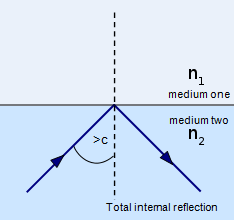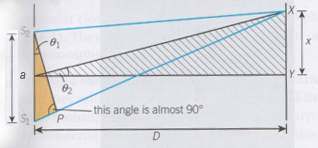Waves Revision

# Wave equations

As is always the case in Physics, there are plenty of wave equations you need to know for the exam!

## v=fλ

velocity (m/s) = frequency (Hz) x Wavelength (m)

No explanation necessary - just plug in the right numbers.

## f=1⁄T

frequency(Hz) = 1Wave period (s)

The period of oscillation is the amount of time it takes for a whole wavelength to pass a point.

## Refractive index: n=c⁄v

refractive index = speed of light in a vacuumspeed of light in the material

As explained elsewhere, every material has a refractive index - which is a ratio of the speed of light through a material as compared to the speed through a vacuum.

## Critical angle: sinC=n1⁄n2

critical angle = refractive index of outside materialrefractive index of inside materialThe critical angle is the minimum angle a ray of light needs to hit the boundary of a material with, in order to totally reflect internally. This equation gives the angle in degrees.

For total internal reflection to be possible, the refractive index of n1 must be lower than that of n2

## Double slit formula: λ=ax⁄D

wavelength = slit separation x fringe separationdistance to screenThis equation applies to Young's double slit experiment on the diffraction patterns page.